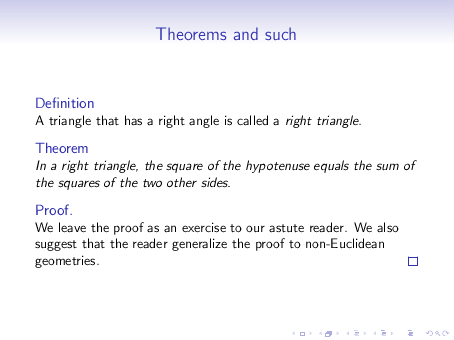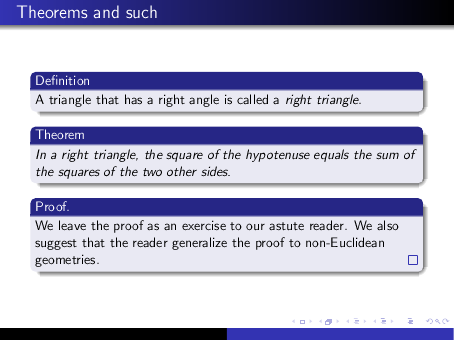# 16  Theorems and such

In Beamer the following LaTeX environments are predefined:

• theorem

• corollary

• definition

• example

• proof

The Beamer theme controls how this environments are rendered. Here is an example:

% theorems-demo-1.tex
\documentclass{beamer}
\usetheme{Singapore}
\begin{document}

\begin{frame}{Theorems and such}

\begin{definition}
A triangle that has a right angle is called
a \emph{right triangle}.
\end{definition}

\begin{theorem}
In a right triangle, the square of the hypotenuse
equals the sum of the squares of the two other sides.
\end{theorem}

\begin{proof}
We leave the proof as an exercise to our astute reader.
We also suggest that the reader generalize the proof to
non-Euclidean geometries.
\end{proof}

\end{frame}
\end{document}If we change the theme to \usetheme{Warsaw}, the slide changes to:These environments are actually defined in the amsmath package which is loaded automatically into Beamer. Therefore the extensive amsmath facilities are available for customizing them. See the documentation in amsthdoc.dvi that comes with the AMS LaTeX extension packages.9

The standard LaTeX \newtheorem command may be used to extend the available theorem-like environments. For instance, if you put:

\newtheorem{proposition}{Proposition}


in the preamble, then you can say:

\begin{proposition}
...
\end{proposition}


9 On the department’s machine, this file is located in the directory:

/usr/share/texmf/doc/latex/amscls/

You can view the file using the command:

xdvi  /usr/share/texmf/doc/latex/amscls/amsthdoc.dvi &